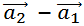reasoning (43) Maths (40) Home (8) integration (6)

## SHORTEST DISTANCE BETWEEN TWO LINES

1st of all we shall find out shortest distance between two Parallel lines.

## Problem 1

Consider two parallel lines whose equations in vector form are given by

Now comparing these equations with standard form , and write

andvectors ,we get

Now applying this formula to find the shortest distance between two lines .
As it is clear from formula , we have to find cross product ofandand then magnitude of vector=√(81)+(196)+(16)
=√293
Magnitude of= √49
= 7
Putting all these values in  the formula of Shortest Distance between two lines .
Now Find distance between two skew lines i.e. Lines which are not Parallel lines.

## Problem 2

Consider two parallel lines whose equations in vector form are given by
Now comparing these equations with standard form , and write

Now applying this formula to find the shortest distance between two lines

Now find cross product and then  magnitude of these two vectors

Don't Forget Watch this Video to understand better

## Appeal

If you are a mathematician Don't forget to visit my Mathematics You tube channel , Mathematics Website and Mathematics Facebook Page , whose links are given below

Share: